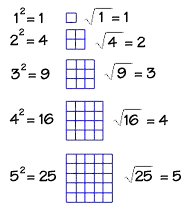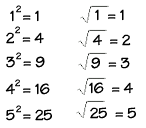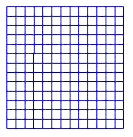Home    |    Teacher    |    Parents    |    Glossary    |    About UsAny number raised to the power of 2 can be modeled using a polygon--the square! That's why we call raising a number to the second power "squaring the number." The perfect squares are squares of whole numbers. Here are the first five perfect squares.

We've shown a geometric model to verify each of these squares. The square root of a number n is a number that, when multiplied by itself, equals n. Here are the square roots of the perfect squares above.This model shows the number 169 as a square. From the model, what is the square root of 169? We can count the number of units making up each side of the square. We find 13 units to a side, so 13 is the square root of 169.Homework Help | Geometry | Relations and SizesEmail this page to a friendSearch·  Congruent figures·  Similar figures·  Squares and     square roots·  Right triangle     factsFirst Glance In Depth Examples WorkoutSquares and square roots